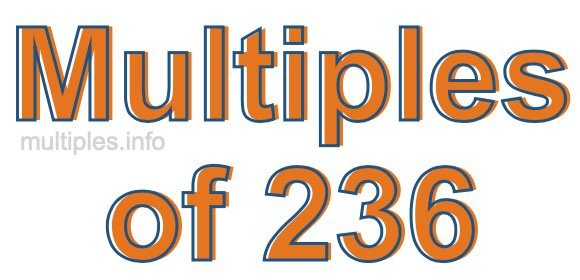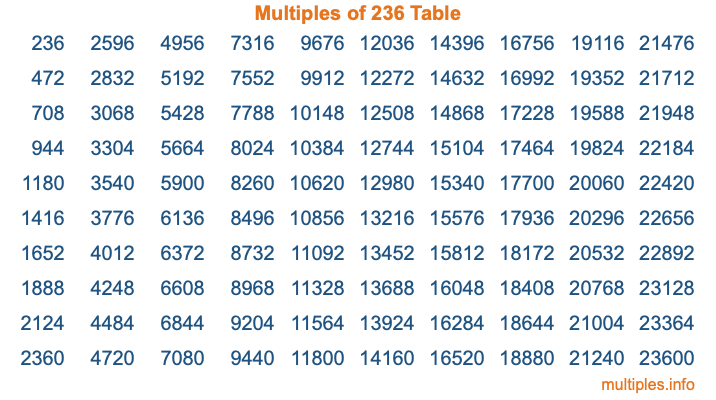Multiples of 236Welcome to the Multiples of 236 page. Here we will first teach you everything you will ever need to know about the multiples of 236, and then give you a study guide summary of everything we taught you to make sure you remember it all. Use this page to look up facts and learn information about the multiples of 236. This page will make you a multiples of two hundred thirty-six expert!

Definition of Multiples of 236
Multiples of 236 are all the numbers that when divided by 236 equal an integer. Each of the multiples of 236 are called a multiple. A multiple of 236 is created by multiplying 236 by an integer.

Therefore, to create a list of multiples of 236, you start with 1 multiplied by 236, then 2 multiplied by 236, then 3 multiplied by 236, and so on for as long as you want. Thus, the list of the first five multiples of 236 is 236, 472, 708, 944, and 1180. To see a larger list of multiples of 236, see the printable image of Multiples of 236 further down on this page. We also have a category where you can choose any nth multiple of 236.

Multiples of 236 Checker
The Multiples of 236 Checker below checks to see if any number of your choice is a multiple of 236. In other words, it checks to see if there is any number (integer) that when multiplied by 236 will equal your number. To do that, we divide your number by 236. If the the quotient is an integer, then your number is a multiple of 236.

Is  a multiple of 236?

Least Common Multiple of 236 and ...
A Least Common Multiple (LCM) is the lowest multiple that two or more numbers have in common. This is also called the smallest common multiple or lowest common multiple and is useful to know when you are adding our subtracting fractions. Enter one or more numbers below (236 is already entered) to find the LCM.

Check out our LCM Calculator if you need more details about the Least Common Multiple or if you need the LCM for different numbers for adding and subtraction fractions.

nth Multiple of 236
As we stated above, 236 is the first multiple of 236, 472 is the second multiple of 236, 708 is the third multiple of 236, and so on. Enter a number below to find the nth multiple of 236.

th multiple of 236

Multiples of 236 vs Factors of 236
236 is a multiple of 236 and a factor of 236, but that is where the similarities end. All postive multiples of 236 are 236 or greater than 236. All positive factors of 236 are 236 or less than 236.

Below is the beginning list of multiples of 236 and the factors of 236 so you can compare:

Multiples of 236: 236, 472, 708, 944, 1180, etc.

Factors of 236: 1, 2, 4, 59, 118, 236

As you can see, the multiples of 236 are all the numbers that you can divide by 236 to get a whole number. The factors of 236, on the other hand, are all the whole numbers that you can multiply by another whole number to get 236.

It's also interesting to note that if a number (x) is a factor of 236, then 236 will also be a multiple of that number (x).

Multiples of 236 vs Divisors of 236
The divisors of 236 are all the integers that 236 can be divided by evenly. Below is a list of the divisors of 236.

Divisors of 236: 1, 2, 4, 59, 118, 236

The interesting thing to note here is that if you take any multiple of 236 and divide it by a divisor of 236, you will see that the quotient is an integer.

Multiples of 236 Table
Below is an image of the first 100 multiples of 236 in a table. The table is in chronological order, column by column. The first column has the first ten multiples of 236, the second column has the next ten multiples of 236, and so on.The Multiples of 236 Table is also referred to as the 236 Times Table or Times Table of 236. You are welcome to print out our table for your studies.

Negative Multiples of 236
Although not often discussed or needed in math, it is worth mentioning that you can make a list of negative multiples of 236 by multiplying 236 by -1, then by -2, then by -3, and so on, to get the following list of negative multiples of 236:

-236, -472, -708, -944, -1180, etc.

Multiples of 236 Summary
Below is a summary of important Multiples of 236 facts that we have discussed on this page. To retain the knowledge on this page, we recommend that you read through the summary and explain to yourself or a study partner why they hold true.

There are an infinite number of multiples of 236.

A multiple of 236 divided by 236 will equal a whole number.

236 divided by a factor of 236 equals a divisor of 236.

The nth multiple of 236 is n times 236.

The largest factor of 236 is equal to the first positive multiple of 236.

236 is a multiple of every factor of 236.

236 is a multiple of 236.

A multiple of 236 divided by a divisor of 236 equals an integer.

236 divided by a divisor of 236 equals a factor of 236.

Any integer times 236 will equal a multiple of 236.

Multiples of a Number
Here you can get the multiples of another number, all with the same attention to detail as we did for multiples of 236 on this page.

Multiples of
Multiples of 237
Did you find our page about multiples of two hundred thirty-six educational? Do you want more knowledge? Check out the multiples of the next number on our list!